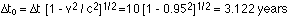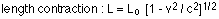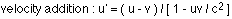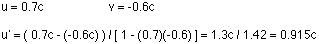## Special relativity

4-10-98

Relevant sections in the book : 28.1 - 28.7

### Physics close to the speed of light

Special relativity, developed by Albert Einstein, applies to situations where objects are moving very quickly, at speeds near the speed of light. Generally, you should account for relativistic effects when speeds are higher than 1 / 10th of the speed of light.

Relativity produces very surprising results. We have no experience dealing with objects traveling at such high speeds, so perhaps it shouldn't be too surprising that we get surprising results. These are a few of the things that happen at relativistic speeds:

• Moving clocks run slow.
• Lengths contract when traveling at high speeds.
• Two events that occur simultaneously for one observer are not simultaneous for another observer in a different frame of reference if the events take place in different locations.

That might sound very odd, but it only sounds odd because we don't see these effects in our daily lives.

### Frames of reference

Everything is relative; it depends on your frame of reference. Different observers see different things if they are in different reference frames (i.e., they are moving relative to each other). Special relativity deals with observers moving at constant velocity; this is a lot easier than general relativity, in which observers can accelerate with respect to each other. Note that frames of reference where the velocity is constant are known is inertial frames.

### Postulates of special relativity

Relativity is based on two very simple ideas; everything else follows from these. These are:

1. The relativity postulate : the laws of physics apply in every inertial reference frame.
2. The speed of light postulate : The speed of light in vacuum is the same for any inertial reference frame (c = 3.00 x 108 m/s). This is true no matter how fast a light source is moving relative to an observer.

### Time dilation

Time dilation refers to the fact that clocks moving at close to the speed of light run slow. Consider two observers, each holding an identical clock. These clocks work using pulses of light. An emitter bounces light off a mirror, and the reflected pulse is picked up by a detector next to the emitter. Every time a pulse is detected, a new pulse is sent out. So, the clock measures time by counting the number of pulses received; the interval between pulses is the time it takes for a pulse to travel to the mirror and back.

If our two observers are stationary relative to each other, they measure the same time. If they are moving at constant velocity relative to each other, however, they measure different times. As an example, let's say one observer stays on the Earth, and the other goes off in a spaceship to a planet 9.5 light years away. If the spaceship travels at a speed of 0.95 c (95% of the speed of light), the observer on Earth measures a time of 10 years for the trip.

The person on the spaceship, however, measures a much shorter time for the trip. In fact, the time they measure is known as the proper time. The time interval being measured is the time between two events; first, when the spaceship leaves Earth, and second, when the spaceship arrives at the planet. The observer on the spaceship is present at both locations, so they measure the proper time. All observers moving relative to this observer measure a longer time, given by:In this case we can use this equation to get the proper time, the time measured for the trip by the observer on the spaceship:So, during the trip the observer on Earth ages 10 years. Anyone on the spaceship only ages 3.122 years.

It is very easy to get confused about who's measuring the proper time. Generally, it's the observer who's present at both the start and end who measures the proper time, and in this case that's the person on the spaceship.

### Length contraction

Carrying on with our example of the spaceship traveling to a distant planet, let's think about what it means for measuring distance. The one thing that might puzzle you is this: everything is relative, so a person on the Earth sees the clock on the spaceship running slow. Similarly, the person on the Earth is moving at 0.95c relative to the observer on the spaceship, so the observer on the ship sees their own clock behaving perfectly and the clock on the Earth moving slow. So, if the clock on the spaceship is measuring time properly according to an observer moving with the clock, how can we account for the fact that the observer on the ship seems to cover a distance of 9.5 light years in 3.122 years, which would imply that they're traveling at a speed of 3.04c?

That absolutely can not be true. For one thing, one of the implications of relativity is that nothing can travel faster than c, the speed of light in vacuum. c is the ultimate speed limit in the universe. For another, two observers will always agree on their relative velocities. If the person on the Earth sees the spaceship moving at 0.95c, the observer on the spaceship agrees that the Earth is moving at 0.95c with respect to the spaceship (and because the other planet is not moving relative to the Earth), everyone's in agreement that the relative velocity between the spaceship and planet is 0.95c.

So, distance is velocity multiplied by time and we know the velocity and time measured by the observer on the spacecraft is 0.95c and 3.122 years. This implies that they measure a distance for the trip of 2.97 light-years, much smaller than the 9.5 light-year distance measured by the observer on the Earth.

This is in fact exactly what happens; a person who is moving measures a contracted length. In this case, the person on the Earth measures the proper length, because they are not moving relative to the far-off planet. The observer on the spaceship, however, is moving relative to the Earth-planet reference frame, so they measure a shorter distance for the distance from the Earth to the planet. The length measured by the moving observer is related to the proper length by the equation:In this case we can solve for the length measured by the observer on the spaceship:This agrees with what we calculated above, as it should.

One important thing to note about length contraction: the contraction is only measured along the direction parallel to the motion of the observer. No contraction is seen in directions perpendicular to the motion.

### Relative velocities

At the start of PY105 we talked about relative velocities. Let's say you're standing on an interstate freeway that runs north and south. You see a truck heading north at 60 km/h, and a car heading south at 70 km/h. All three of you (you, the car driver, and the truck driver) agree on these points:

• the truck is traveling at 60 km/h north relative to you, and 130 km/h north relative to the car.
• the car is traveling at 70 km/h south relative to you, and 130 km/h south relative to the truck.
• you are traveling at 60 km/h south relative to the truck, and 70 km/h north relative to the car.

Two observers always agree on their relative velocity. The simple addition we used to get the velocity of the truck relative to the car can not be applied to a relativistic situation, however.Let's say you now stand on an intergalactic freeway. You see a truck heading in one direction at 0.6c, and a car heading in the opposite direction at 0.7c. What is the velocity of the truck relative to the car? It is not 1.3c, because nothing can travel faster than c. The relative velocity can be found using this equation:In this case, u is the velocity of the car relative to you, v is the velocity of the truck relative to you, and u' is the velocity of the car relative to the truck. Taking the direction the car is traveling to be the positive direction:So, now everyone involved agrees on this:

• the truck is traveling at 0.6c relative to you, and 0.915c relative to the car.
• the car is traveling at 0.7c relative to you, and 0.915c relative to the truck.
• you are traveling at 0.6c relative to the truck, and 0.7c relative to the car.

The relativistic equation for velocity addition shown above can also be used for non-relativistic velocities. We're more used to adding velocities like this : u' = u - v. This is exactly what the relativistic equation reduces to for velocities much less than the speed of light. The relativistic equation applies to any situation; the one we're used to is a special case that applies only for small velocities.